## Páginas

### Coherence induced by a train of ultrashort pulses in a Lambda-type system

Publicações -  Coherence induced by ...

1. Introduction
2. Numerical results
3. Analytical approach
5. Conclusions

We present an analysis of the coherent population trapping in a three-level Lambda system when excited by an ultrashort pulse train near the one- and two-photon resonances. Numerical results using Bloch equations give the transient response of the system and clearly reveal a comb of electromagnetically induced transparency (EIT) lines. An analytical solution to describe the EIT lines is derived in the steady-state regime and in the weak field limit. Moreover, numerical results indicate that selective velocity spectroscopy makes possible the observation of the comb of EIT lines in Doppler broadening media.

1. Introduction

The optical frequency comb  generated by mode-locked femtosecond lasers has played an important role in various fields such as optical frequency metrology  and quantum coherent control . Recently, new proposals in the field of quantum information science have emerged , such as the implementation of a quantum logic gate controlled through the phase relationship between successive pulses . Most of the applications explore the perfect periodicity of the frequency comb in addition to the fact that one can tune the repetition rate of the mode-locked laser. In particular, when the repetition rate, or its multiples, matches the splitting between the two lower states in a Lambda-type system, a coherent population trapping (CPT)  is induced. In this context, a very special case is the electromagnetically induced transparency (EIT) , i.e., the system is made transparent to a resonant probe field.

EIT in the ultrashort pulse regime was theoretically predicted by Kocharovskaya and Khanin ; in fact, they showed that, in the steady-state regime, a train of ultrashort pulses may also induce coherent population trapping when interacting with a three-level Lambda system. More recently, an analytic iterative treatment was applied to study the transient behavior in a degenerate Lambda system . The experimental observation of the EIT signal as a function of the repetition rate of an ultrashort pulse train produced by a mode-locked diode laser was described by Sautenkov et al. . Moreover, the repetition rate spectroscopy was explored by Arissian and Diels  using a picosecond mode-locked laser.

In this article, we present an analytical solution for the coherence induced by a long train of ultrashort optical pulses in a three-level lambda system, near the conditions of one- and two-photon resonances. The solution is obtained in the frequency domain and it is valid for the stationary regime and in the weak field limit. Two assumptions are important. One is related to the fact that a mode-locked laser generates an infinity train of very regular ultrashort pulses that, in the frequency domain, gives a comb of narrow lines which can be written as delta functions. The other is related to the atomic system, for which all the atomic relaxation times are considered longer than the laser repetition period. In this case, the atom interacts not only with the spectrum of a single pulse but with the frequency comb of the pulse train; in fact, the effect is similar to that of an atom interacting with an infinity number of cw lasers whose frequency separation is equal to the repetition rate and having zero relative phases. This behavior can be understood using a physical picture, analogous to the Ramsey fringes, where constructive and destructive interferences, due to the time-delayed phases acquired with the succession of the pulses, occur during the atomic relaxation times. This description allows us to make comparisons with EIT results obtained using cw lasers.

Before discussing the stationary regime, we analyze the temporal evolution of the atomic population and coherence as a function of the laser field intensity, and the number of driving pulses in the excitation train. The interaction of each pulse with the atomic system is described by the optical Bloch equations and the response of the medium, obtained by a direct numerical integration, is compared with our analytical results.

Our treatment for the problem is based on the scheme of Fig. 1(a). We consider a three-level atom in a Lambda configuration interacting with a train of ultrashort pulses generated by a mode-locked laser. Following Ref.  and taking into account the pulse-to-pulse phase shift, the total electric field for the pulse train can be written as:
The coupling between the atom and the electric field reads:
The Bloch equations describing the temporal evolution of the elements of the atomic density matrix are given by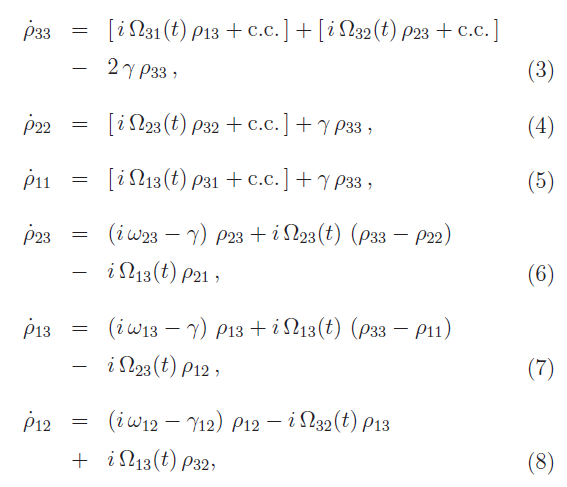We consider that the transition between the two lower states is dipole-forbidden and that the spontaneous decay rate of the excited state is smaller than the pulse repetition rate. In this case, the atom could never completely relax between two consecutive pulses, leading to accumulation in both population and coherence . In the frequency domain, this means that the atomic system can distinguish between two neighboring modes of the frequency comb.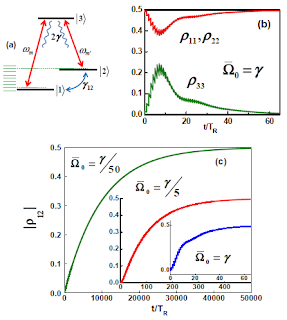Fig. 1

Three resonance situations are investigated and the conditions at which the atomic system reaches a stationary state, for each case, are obtained by numerical integration of the Bloch equations using a standard fourth-order Runge-Kutta method. Optical pumping occurs when a mode of the frequency comb matches only one-photon transition . In this case, no net population is obtained at the excited state and the coherence between the two lower levels, evolves to zero. The Raman resonance is characterized by a pure two-photon transition, and it is achieved whenever the pulse repetition rate or its multiples coincides with the frequency difference between the two lower states . However, the more interesting situation takes place when both one- and two-photon resonances occur simultaneously, which corresponds to the EIT condition. In this case, the stationary regime of maximum coherence is achieved for a number of driving pulses much smaller than that needed for maximum coherence in Raman resonance.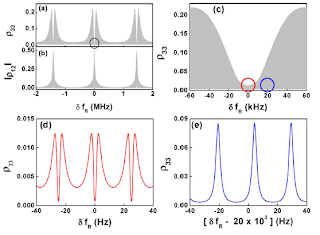Fig. 2

Temporal evolution of the populations, during the interaction with a train of pulses, when both one- and two-photon resonances occur simultaneously, is shown in Fig. 1(b). The results are similar to those obtained for a degenerate Lambda system using an analytic iterative solution . To simplify the numerical computation we modeled the pulses as having square envelopes with a time duration of 100 fs. We have also considered µ13 = µ23 = µ and defined an average Rabi frequency,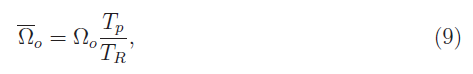In these resonance conditions the coherence between the two lower states, is showed in Fig. 1(c). Whereas for weak fields full coherence is reached only after the interaction with more than fifty thousand pulses, full coherence is obtained before one hundred driving pulses and Rabi oscillations (due to coherent accumulation) are observed in both coherence and population .

In the stationary regime, the atomic response to the excitation by a train of femtosecond (fs) pulses can be studied as a function of the pulse repetition rate. Figure 2 shows (a) the excited state population, and (b) the coherence, as a function of repetition rate. The results were obtained for the same parameters of Fig. 1, after the interaction of the atomic system with more than five hundred driving pulses. The maximum values of the excited state population are modulated by the Raman resonances of the medium. As shown in Fig. 2(a), an increase of maximum values of the excited state population is always observed as repetition rate approaches the two-photon resonance. However, exactly at the two-photon resonance, its goes to zero [Fig. 2(c)] and the envelope of the coherence reaches its maximum value [Fig. 2(b)]. The dip in the Raman resonance and the maximum value of the coherence envelope are signatures of the EIT window. The experimental observation of these EIT windows, using ultrashort pulses, was reported in Refs. [10, 11]. In Figs. 2(d) and (e) we have a zoom of the two regions inside the Raman resonance. We can clearly see the peaks in the population due to the one-photon resonance condition.

3. Analytical approach

The strong dependence on the modes of the frequency comb of the atomic population and coherence, as indicated in Fig. 2, can be better understood if, instead of work in the time domain, we look at the frequency domain . We are interested in the atomic response near the two-photon resonance, and after a long time interaction with the pulse train as the system reaches the steady-state regime. In the weak pulse limit we can neglected the variations in the populations.

We began our treatment in the frequency domain by taking the Fourier transform (FT) of the pulse train :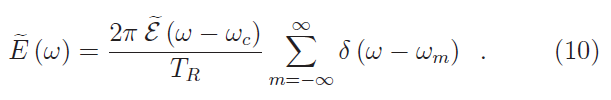From Eq. (10) we clearly see that the frequency spectrum consist of a comb of very narrow lines.

Using the above-mentioned values for the populations and taking the FT of each term of Eqs. (6)-(8), we can write the atomic coherence, in the frequency domain, as: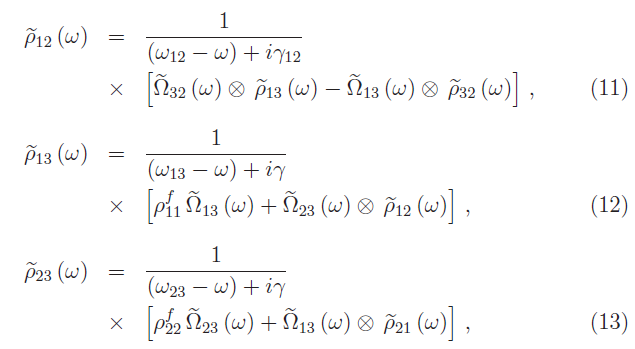where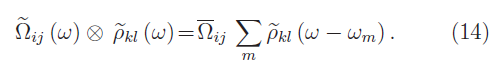We have also considered that all modes of the comb, near the one- and two-photon transitions, have the same
amplitude. This allows us to write an average Rabi frequency for each transition i-j: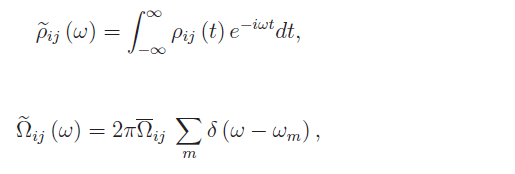Using Eq. (10) and the convolution theorem, we can write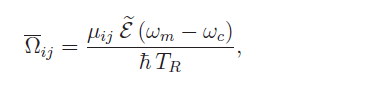In the weak field limit, we can neglect the processes involving resonances of four or more photons and then obtain an analytical expression for the coherence between the two lower states, which in the time domain, is written as (see Appendix A):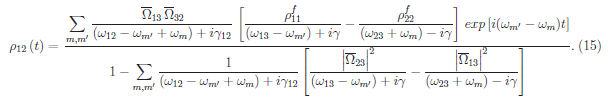The denominator in Eq. (15) indicates that we select only processes involving two modes of frequency comb. When the frequency difference of these two modes, is equal to the splitting between the two lower states, the atomic system is pumped into a superposition of the two states, and thereby the increase of the coherence characterizes the EIT condition. In this situation, we also have the contribution of one-photon resonance from each mode, as indicated by the denominators. This can be clearly seen in Fig. 3, where the coherence| is plotted as a function of the pulse repetition rate. The envelope of the maximum values of the coherence, determines the EIT window and each peak corresponds to an one-photon resonant transition. What we see is the EIT window with a comb of very narrow EIT lines.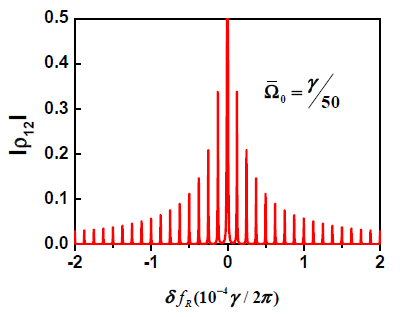Fig. 3

We can also use Eq. (15) to obtain the linewidth of the EIT window that modulate the comb of EIT lines. For this, we keep each branch of the Lambda transition on resonance and study the variation of coherence over the two-photon resonance (see Appendix B). In the simplest case of equal Rabi frequencies, we find that the EIT window linewidth, can be written as: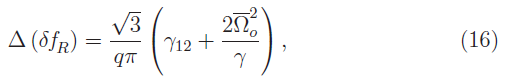where q is the number of modes between the two lower states. Eq. (16) gives the same behavior as obtained in the case of CPT with cw lasers . The first term is due to the relaxation rate of the coherence, whereas the second term, proportional to the field intensity, describes the power broadening.

In Figs. 4(a)-(c) we plot the envelope coherence a function of repetition rate in order to compare the linewidth of the EIT window obtained from our analytical approach, Eq. (15), with the numerical results calculated from the Bloch equations, Eqs. (3)-(8), for three different values of the average Rabi frequency. For very low field intensity, where the analytical expression is valid, the two curves overlap. For strong fields, the analytical approach is not longer valid, and thus the numerical results show a narrower window due to saturation effects.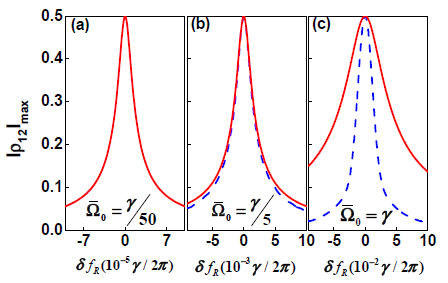Fig. 4
We also remark that EIT window, as described by the envelope coherence in Fig. 4, was observed with ultrashort pulses train produced by a mode locked diode laser , and with a picosecond laser . In the latter experiment the fluorescence of the excited state was detected and a decrease of the excited state was observed, corresponding to the dip in the Raman resonance as shown in Fig. 2(c).

As we see in Fig. 3, the excitation of a Lambda-type system by a train of ultrashort pulses reveals a series of very narrow EIT lines inside the EIT window. As described before, each narrow EIT line occurs when the system is simultaneously in one- and two-photon resonances [Fig.2(d)]. However, when we analyze a Doppler broadening atomic sample, for each value of repetition rate we need to integrate out the atomic response over the Doppler profile, so the one-photon resonances are blurred and only the dip in the Raman resonance (the EIT window) is observed . To distinguish the one-photon resonances in a Doppler broadening vapor, we can fix the pulse repetition rate and use the velocity selective spectroscopy to probe the atomic response. The variation of the lower state population, in the Doppler profile, is shown in Fig. 5. The numerical results were obtained from Eqs. (3)-(8), for both transitions and after the interaction of the atomic system with one thousand driving pulses. The other parameters are equal to those of Fig. 2. In Fig. 5(a) the repetition rate is equal to 100 MHz, corresponding to the situation where all the atoms are at the two-photon resonance, but only groups of atoms with specific velocities will also be at the one-photon resonance and thereby manifest the EIT effect.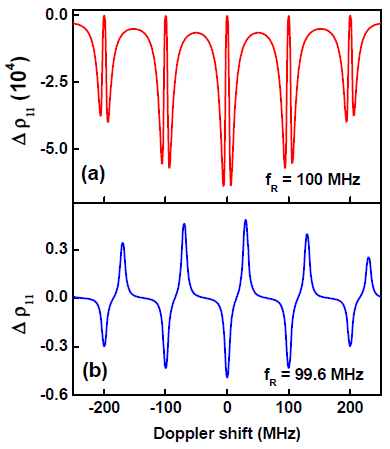Fig. 5
Lastly, when we change the pulse repetition rate for a value distinct from an harmonic of energy between two ground states, as shown in Fig. 5(b), we observe only the peaks corresponding to the population transfer processes due to the one-photon resonances in each leg of the lambda system for different groups of atoms. The situation described in Fig. 5(b) has been detailed investigated, as a function of the atomic density and laser intensities, for both lines, D1 and D2, of Rb alkaline metal [20, 21]. Moreover, the results showed in Fig. 5(a) indicate that the selective velocity spectroscopy can be used to observe the comb of the EIT lines in the Doppler profile of an inhomogeneously broaden medium.

In this paper we have presented a study of the coherent population trapping in a three-level Lambda system excited by an ultrashort pulse train near the one- and two-photon resonances. The temporal evolution of the atomic system and the response to a repetition rate spectroscopy were obtained from the numerical integration of the Bloch equations. The results showed the occurrence of a comb of very narrow EIT lines, determined by the one-photon resonances, that appears inside the EIT windows. We also worked in the frequency domain to explore a more intuitive physical picture. In this context, we derived a closed analytical solution to describe these EIT signals, which is valid in the weak field regime. In particular, we showed that the linewidth of the EIT window can be modeled by two cw lasers with equal intensities. Further, a comparison of the analytical results with those obtained by a direct numerical integration of the Bloch equations was also present. Finally, the numerical results indicate that selective velocity spectroscopy appears as very useful tool to observe the comb of EIT lines in Doppler broadening media.

Appendix A: Derivation of Eq. (15)

Using Eq. (14) to write the convolutions, we obtain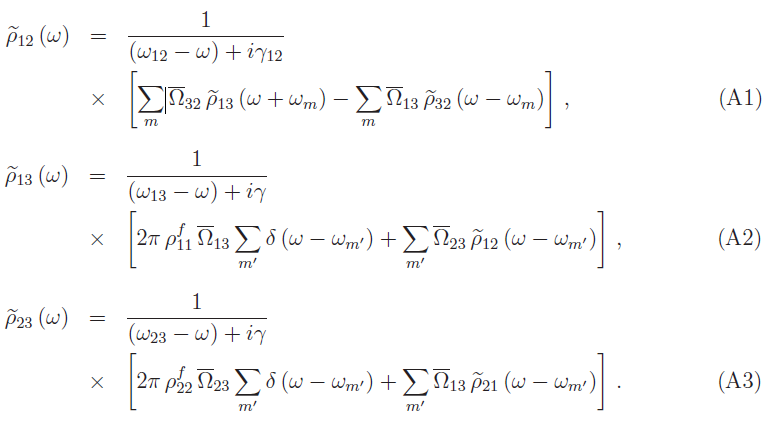As we are interested in the coherence between the two lower states, we use Eqs. (A2)-(A3) into Eq. (A1). We find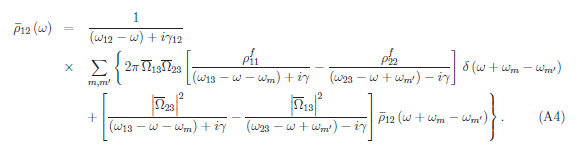Eq. (A4) allows an iterative solution fo rcoherence in frequency domain. A closed solution is obtained in the
weak field limit, when only two-photon resonances are considered. In this case, we obtain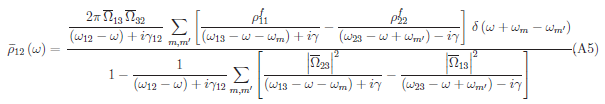So, the coherence between the two lower states, in the stationary regime, is given by the FT of Eq. (A5) and is displayed in Eq. (15).

Appendix B: Derivation of Eq. (16)

In order to derive the linewidth of the EIT window we consider only the two modes (m and m' ) of the comb frequency which are near the Raman resonance. By assuming one-photon resonances, we can write Eq. (15) as: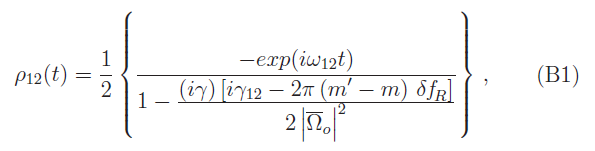The maximum value for the coherence is achieved at the two-photon resonance: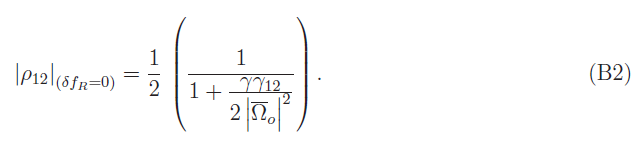Acknowledgments

We acknowledge helpful discussions with D. Felinto and L. H. Acioli. This work was supported by the Brazilian agencies CNPq, FACEPE and CAPES.

References

1. Th. Udem, R. Holzwarth, and T. W. H¨ansch, “Optical frequency metrology,” Nature (London) 416, 233–236 (2002).
2. J. Ye, and S. T. Cundiff, Femtosecond Optical Frequency Comb Technology: Principle, Operation and Application (Springer, New York, USA, 2005).
3. M. C. Stowe, A. Pe’er, and J. Ye, “Control of four-level quantum coherence via discrete spectral shaping of an optical frequency comb,” Phys. Rev. Lett. 100, 203001 (2008).
4. G. Campbell, A. Ordog, and A. I. Lvovsky, “Multimode electromagnetically induced transparency on a single atomic line,” New J. Phys. 11, 103021 (2009).
5. D. Hayes, D. N. Matsukevich, P. Maunz, D. Hucul, Q. Quraishi, S. Olmschenk, W. Campbell, J. Mizrahi, C. Senko, and C. Monroe, “Entanglement of atomic qubits using an optical frequency comb,” Phys. Rev. Lett. 104, 140501 (2010).
6. E. Arimondo, “Coherent population trapping in laser spectroscopy,” Progr. Opt. 35, 257–355 (1996).
7. M. Fleischhauer, A. Imamoglu, J. P. Marangos, “Electromagnetically induced transparency: optics in coherent media,” Rev. Mod. Phys. 77, 633–673 (2005).
8. O. A. Kocharovskaya, and Ya. I. Khanin, “Population trapping and coherence bleaching of a three-level medium by a periodic train of ultrashort pulses,” Sov. Phys. JETP 63, 945–950 (1986).
9. A. A. Soares, and L. E. E. de Araujo, “Coherent accumulation of excitation in the electromagnetically induced transparency of an ultrashort pulse train,” Phys. Rev. A 76, 043818 (2007).
10. V. A. Sautenkov, Y. V. Rostovtsev, C. Y. Ye, G. R. Welch, O. Kocharovskaya, and M. O. Scully, “Electromagnetically induced transparency in rubidium vapor prepared by a comb of short optical pulses,” Phys. Rev. A 71, 063804 (2005).
11. L. Arissian, and J.-C. Diels, “Repetition rate spectroscopy of the dark line resonance in rubidium,” Opt. Commun. 264, 169–173 (2006).
12. S. T. Cundiff, “Phase stabilization of ultrashort optical pulses,” J. Phys. D: Appl. Phys. 35, R43–R59 (2002).
13. D. Felinto, C. A. C. Bosco, L. H. Acioli, and S. S. Vianna, “Coherent accumulation in two-level atoms excited by a train of ultrashort pulses,” Opt. Commun. 215, 69–73 (2003).
14. D. Aumiler, T. Ban, H. Skenderovi´c, and G. Pichler, “Velocity Selective Optical Pumping of Rb Hyperfine Lines Induced by a Train of Femtosecond Pulses,” Phys. Rev. Lett. 95, 233001 (2005).
15. J. Mlynek, W. Lange, H. Harde, and H. Burggraf, “High-resolution coherence spectroscopy using pulse trains,” Phys. Rev. A 24, 1099–1102 (1981).
16. T. H. Yoon, A. Marian, J. L. Hall, and J. Ye, “Phase-coherent multilevel two-photon transitions in cold Rb atoms: Ultrahigh-resolution spectroscopy via frequency-stabilized femtosecond laser,” Phys. Rev. A 63, 011402(R) (2000).
17. R. M. Whitley, and C. R. Stroud, Jr, “Double optical resonance,” Phys. Rev. A 14, 1498-1513 (1976).
18. J. Vanier, A. Godone, and F. Levi, “Coherent population trapping in cesium: dark lines and coherent microwave emission,” Phys. Rev. A 58, 2345-2358 (1998).
19. D. Aumiler, “Coherent population trapping in 87Rb atoms induced by the optical frequency com excitation,” Phys. Rev. A 82, 055402 (2010).
20. T. Ban, D. Aumiler, H. Skenderovic, S. Vdovic, N. Vujicic, and G. Pichler, “Cancellation of the coherent accumulation in rubidium atoms excited by a train of femtosecond pulses,” Phys. Rev. A 76, 043410 (2007).
21. M. Polo, C. A. C. Bosco, L. H. Acioli, D. Felinto, and S. S. Vianna, “Coupling between cw lasers and a frequency comb in dense atomic samples,” J. Phys. B: At. Mol. Opt. Phys. 43, 055001 (2010).